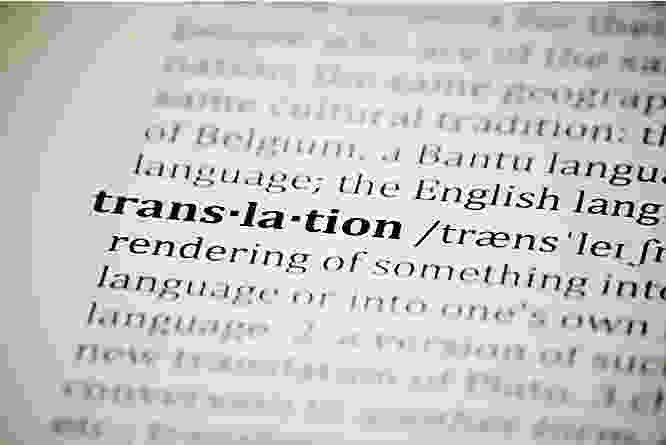•webmaster9757

# Interesting to Think about Word Vectors

March 27, 2018| By Mark Stadtmueller, VP, Product StrategyIn AI, word vectors are very interesting to think about.

In deep neural networks, inputs are put into the network during training and the networks learn to get accurate results by comparing its “answers” with the correct answers and then editing itself to get more accurate.  Once the network is trained to a sufficient accuracy, the network then uses the same types of inputs from new data to deliver results where the correct answers were previously not known.

But, the deep neural network does all this work by making calculations.  In order to do that, the inputs all need to be converted to numbers if they are not already.  So, for instance a picture is read in as pixel values which are numeric.  Words also have to be converted into numbers.  In order to do that, words are converted into “Word Vectors”.  In a word vector, the meaning of the word is encoded into a series of numbers or embeddings, and in a simplistic sense, each number in that vector represents an aspect of that word.  It is akin to describing a movie.  A movie may have traits like being “historical” or “science fiction” or having a certain leading actor.  The same is done with words.  It is even more complicated though as word vectors only work for the context in which the word vectors are created.  So, words in a word vector trained on Shakespeare would have different numeric vector representations than words in a word vector trained on sports articles.

These embeddings/vectors (series of numbers) for each word in a word vector are all the same length, but the person designing the word vector chooses the length.  It is somewhat arbitrary but the word vector designers usually optimize the length, trading off accuracy with training time and compute cost.  Typical lengths (or dimensions) can be from 100 to 1000.  Once you have the word vector (a common one is Google’s word2vec) that is appropriate for the context, then words can be input into a deep neural network.  A common type of deep neural network for natural language processing is a recurrent neural network where a series of words is fed into the network word by word (as word vectors) and the network learns meaning from this stream of word vectors.

So, it gets really interesting, Word Vectors are actually encoding the meaning of a word by a series of numbers.

Essentially, they become numeric dictionaries that deep neural networks use to understand the meaning of a word.  And like dictionaries, the deep neural network needs to understand the context under which a word is being used and the different potential uses of that word.  So, for instance, take the word “help”.  A deep neural network would have to understand the context.  Is it being used in a want ad? Or is it being used in a crisis?  The word vector encodes these potential uses so the deep neural network can learn from it.  And even in an AI blog post, where the word “vector” is being used, the word vector can encode its meaning in a series of numbers.

Tags: Top 10 AI New Years Resolutions

word vectors

deep neural networks

AI

See All